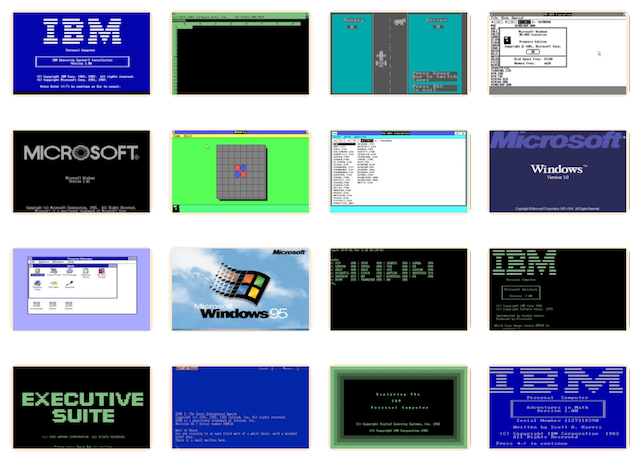# PCjs Machines

Home of the original IBM PC emulator for browsers.## 6502 Overflow Tests

This program from 6502.org verifies 6502 Overflow (V) Flag behavior.

``````;
; The following code comes from:
;
;	http://www.6502.org/tutorials/vflag.html
;
; and is built with:
;
;	ca65 vflag.asm -I ../.. -l -o vflag.obj
;	ld65 vflag.obj -o vflag.bin --target none
;
; An ASCII hex file which the OSI machine-language monitor can load
; is produced with the following commands:
;
;	echo -n ".0200/" > vflag.txt
;	hexdump -e '1/1 "%02X\n"' -v vflag.bin >> vflag.txt
;	echo -n ".0200" >> vflag.txt
;
.include "sim.inc"

; Demonstrate that the V flag works as described
;
; Returns with ERROR = 0 if the test passes, ERROR = 1 if the test fails
;
; Five (additional) memory locations are used: ERROR, S1, S2, U1, and U2
; which can be located anywhere convenient in RAM
;
; The program below takes about 16 seconds to complete at 1 MHz.
;
TEST:	CLD       ; Clear decimal mode (just in case) for test
LDA #1
STA ERROR ; Store 1 in ERROR until test passes
LDA #\$80
STA S1    ; Initalize S1 and S2 to -128 (\$80)
STA S2
LDA #0
STA U1    ; Initialize U1 and U2 to 0
STA U2
LDY #1    ; Initialize Y (used to set and clear the carry flag) to 1
CPX #1
BEQ ERR   ; End if V and unsigned result do not agree (X = 1)
JSR SUB   ; Test SBC
CPX #1
BEQ ERR   ; End if V and unsigned result do not agree (X = 1)
INC S1
INC U1
BNE LOOP  ; Loop until all 256 possibilities of S1 and U1 are tested
INC S2
INC U2
BNE LOOP  ; Loop until all 256 possibilities of S2 and U2 are tested
DEY
BPL LOOP  ; Loop until both possiblities of the carry flag are tested
LDA #0
STA ERROR ; All tests pass, so store 0 in ERROR
SIM_MSG "PASS"
BEQ DONE
ERR:	SIM_MSG "FAIL"
DONE:	SIM_HLT
;
;
; X is initialized to 0
; X is incremented when V = 1
; X is incremented when the unsigned result predicts an overflow
; Therefore, if the V flag and the unsigned result agree, X will be
; incremented zero or two times (returning X = 0 or X = 2), and if they do
; not agree X will be incremented once (returning X = 1)
;
ADD:	CPY #1   ; Set carry when Y = 1, clear carry when Y = 0
LDA S1   ; Test twos complement addition
LDX #0   ; Initialize X to 0
INX      ; Increment X if V = 1
ADD1:	CPY #1   ; Set carry when Y = 1, clear carry when Y = 0
LDA U1   ; Test unsigned addition
BCS ADD3 ; Carry is set if U1 + U2 >= 256
BMI ADD2 ; U1 + U2 < 256, A >= 128 if U1 + U2 >= 128
INX      ; Increment X if U1 + U2 < 128
ADD3:	BPL ADD4 ; U1 + U2 >= 256, A <= 127 if U1 + U2 <= 383 (\$17F)
INX      ; Increment X if U1 + U2 > 383
;
; Test SBC
;
; X is initialized to 0
; X is incremented when V = 1
; X is incremented when the unsigned result predicts an overflow
; Therefore, if the V flag and the unsigned result agree, X will be
; incremented zero or two times (returning X = 0 or X = 2), and if they do
; not agree X will be incremented once (returning X = 1)
;
SUB:	CPY #1   ; Set carry when Y = 1, clear carry when Y = 0
LDA S1   ; Test twos complement subtraction
SBC S2
LDX #0   ; Initialize X to 0
BVC SUB1
INX      ; Increment X if V = 1
SUB1:	CPY #1   ; Set carry when Y = 1, clear carry when Y = 0
LDA U1   ; Test unsigned subtraction
SBC U2
PHA      ; Save the low byte of result on the stack
LDA #\$FF
SBC #\$00 ; result = (65280 + U1) - U2, 65280 = \$FF00
CMP #\$FE
BNE SUB4 ; Branch if result >= 65280 (\$FF00) or result < 65024 (\$FE00)
PLA      ; Get the low byte of result
BMI SUB3 ; result < 65280 (\$FF00), A >= 128 if result >= 65152 (\$FE80)
SUB2:	INX      ; Increment X if result < 65152 (\$FE80)
SUB3:	RTS
SUB4:	PLA      ; Get the low byte of result (does not affect the carry flag)
BCC SUB2 ; The carry flag is clear if result < 65024 (\$FE00)
BPL SUB5 ; result >= 65280 (\$FF00), A <= 127 if result <= 65407 (\$FF7F)
INX      ; Increment X if result > 65407 (\$FF7F)
SUB5:	RTS

.data
ERROR:	.byte	0
S1:		.byte	0
S2:		.byte	0
U1:		.byte	0
U2:		.byte	0

``````